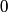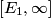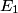## Solution to 1986 Problem 47

 In the Franck-Hertz experiment, a voltage difference accelerates electrons through a glass tube filled with mercury in gaseous form. The apparatus allows one to measure the energy that electrons lose due to collisions with mercury atoms. The conclusion of the experiment is that the energy lost by electrons is discretized in units of the first excitation energy of mercury (the energy of a mercury atoms first excited state). When the potential difference times the magnitude of the electron charge is at least as large as the first excitation energy, the electrons can ionize mercury atoms, which is an inelastic collision. On the other hand, when the potential difference times the magnitude of the electron charge is less than the first excitation energy, this doesn't happen, and the electrons scatter only elastically from mercury atoms. So, both elastic and inelastic collisions are possible, and answers (A) and (B) are both wrong. Answer (D) is wrong because an accelerated electron can also excite the mercury atom to its second, third, or higher excited state, thus losing more energy. Answer (E) is wrong because the energy lost varies continuously, in fact it is constant at$0$, when the potential difference times the magnitude of the electron charge is less than the first excitation energy. That leaves answer (C), which is an accurate statement. The energy range referred to is presumably$[E_1, \infty]$, where$E_1$ is the first excitation energy of mercury. Therefore, answer (C) is correct.